# Algebra Bricks (2)

In this worksheet, students add and subtract expressions to find the missing algebra bricks.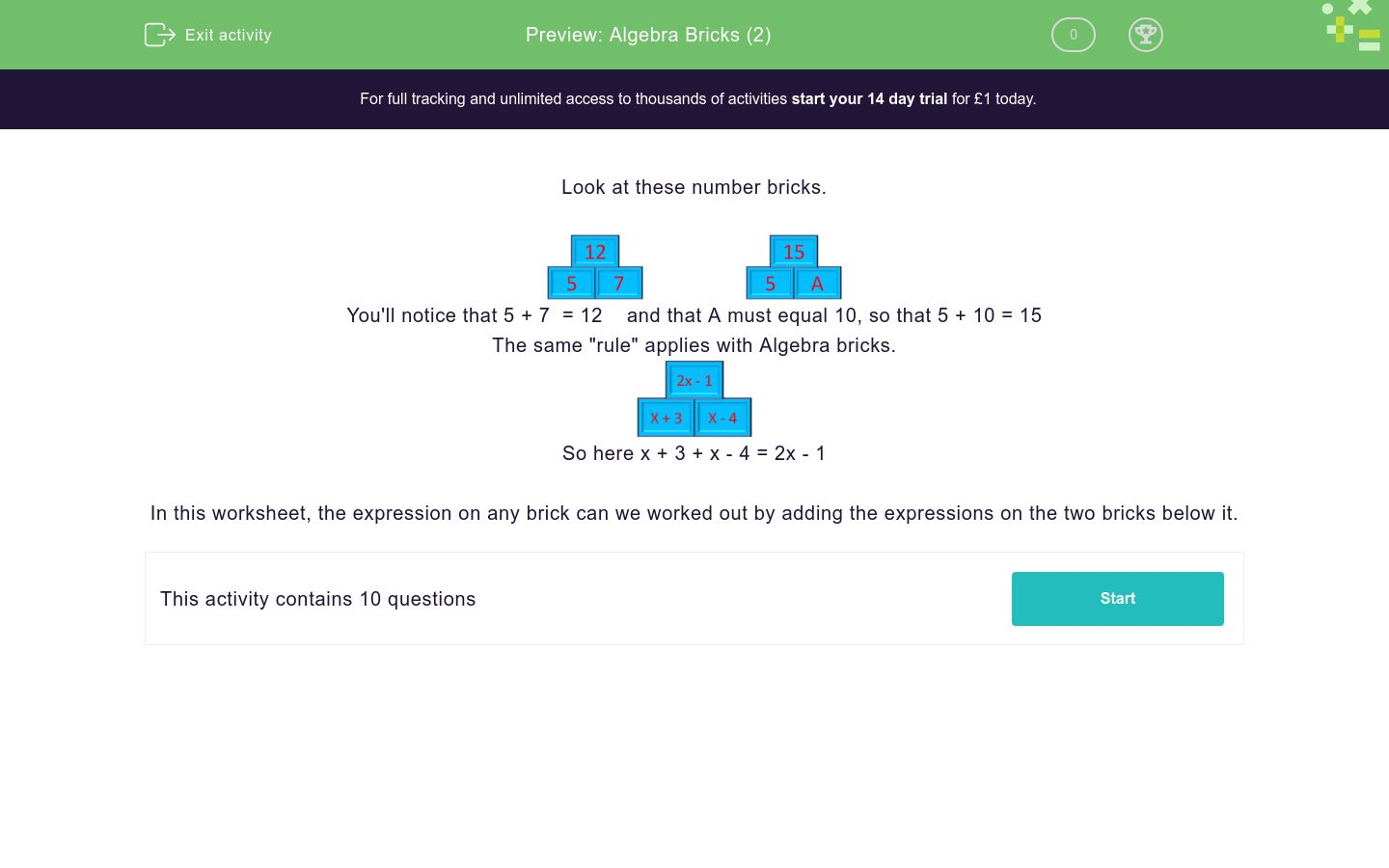Key stage:  KS 3

Curriculum topic:   Algebra

Curriculum subtopic:   Understand Expressions, Equations, Inequalities, Terms and Factors

Difficulty level:### QUESTION 1 of 10

Look at these number bricks.You'll notice that 5 + 7  = 12    and that A must equal 10, so that 5 + 10 = 15

The same "rule" applies with Algebra bricks.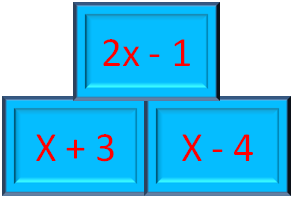So here x + 3 + x - 4 = 2x - 1

In this worksheet, the expression on any brick can we worked out by adding the expressions on the two bricks below it.

Work out the values of A, B and C in these Algebra bricks.

Match the expressions to the correct letter.## Column B

A
x - 1
B
3x
C
2x + 1

Work out the values of A, B and C in these Algebra bricks.

Match the expressions to the correct letter.## Column B

A
x - 4
B
x - 2
C
2x - 6

Work out the values of A, B and C in these Algebra bricks.

Match the expressions to the correct letter.## Column B

A
5x
B
6x + 2
C
x + 2

Work out the values of A, B and C in these Algebra bricks.

Match the expressions to the correct letter.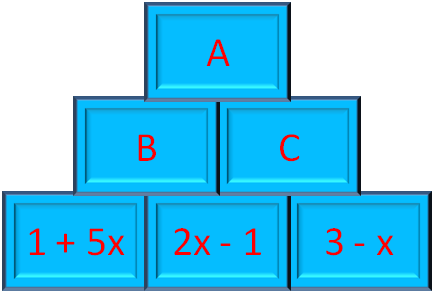## Column B

A
x + 2
B
7x
C
8x + 2

Work out the values of A, B and C in these Algebra bricks.

Match the expressions to the correct letter.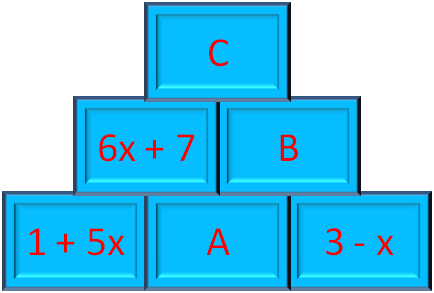## Column B

A
x + 6
B
6x + 16
C
9

Work out the values of A, B and C in these Algebra bricks.

Match the expressions to the correct letter.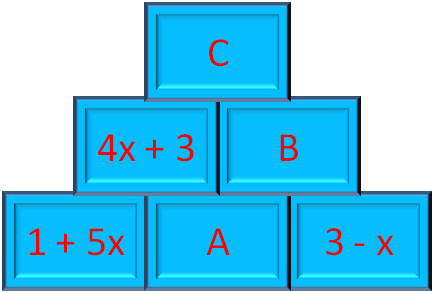## Column B

A
5 - 2x
B
2 - x
C
2x + 8

Work out the values of A, B and C in these Algebra bricks.

Match the expressions to the correct letter.## Column B

A
2x + 11
B
6x + 7
C
4x - 4

Work out the values of A, B and C in these Algebra bricks.

Match the expressions to the correct letter.## Column B

A
2x + 6
B
12x + 4
C
6x + 9

Work out the values of A, B and C in these Algebra bricks.

Match the expressions to the correct letter.## Column B

A
2x + 6
B
5x + 16
C
18x + 46

Work out the values of A, B and C in these Algebra bricks.

Match the expressions to the correct letter.## Column B

A
2x + 6
B
x + 1
C
12x + 20
• Question 1

Work out the values of A, B and C in these Algebra bricks.

Match the expressions to the correct letter.## Column B

A
3x
B
x - 1
C
2x + 1
• Question 2

Work out the values of A, B and C in these Algebra bricks.

Match the expressions to the correct letter.## Column B

A
2x - 6
B
x - 4
C
x - 2
• Question 3

Work out the values of A, B and C in these Algebra bricks.

Match the expressions to the correct letter.## Column B

A
6x + 2
B
5x
C
x + 2
• Question 4

Work out the values of A, B and C in these Algebra bricks.

Match the expressions to the correct letter.## Column B

A
8x + 2
B
7x
C
x + 2
• Question 5

Work out the values of A, B and C in these Algebra bricks.

Match the expressions to the correct letter.## Column B

A
x + 6
B
9
C
6x + 16
• Question 6

Work out the values of A, B and C in these Algebra bricks.

Match the expressions to the correct letter.## Column B

A
2 - x
B
5 - 2x
C
2x + 8
• Question 7

Work out the values of A, B and C in these Algebra bricks.

Match the expressions to the correct letter.## Column B

A
6x + 7
B
2x + 11
C
4x - 4
• Question 8

Work out the values of A, B and C in these Algebra bricks.

Match the expressions to the correct letter.## Column B

A
2x + 6
B
6x + 9
C
12x + 4
• Question 9

Work out the values of A, B and C in these Algebra bricks.

Match the expressions to the correct letter.## Column B

A
2x + 6
B
5x + 16
C
18x + 46
• Question 10

Work out the values of A, B and C in these Algebra bricks.

Match the expressions to the correct letter.## Column B

A
2x + 6
B
x + 1
C
12x + 20
---- OR ----

Sign up for a £1 trial so you can track and measure your child's progress on this activity.

### What is EdPlace?

We're your National Curriculum aligned online education content provider helping each child succeed in English, maths and science from year 1 to GCSE. With an EdPlace account you’ll be able to track and measure progress, helping each child achieve their best. We build confidence and attainment by personalising each child’s learning at a level that suits them.

Get started